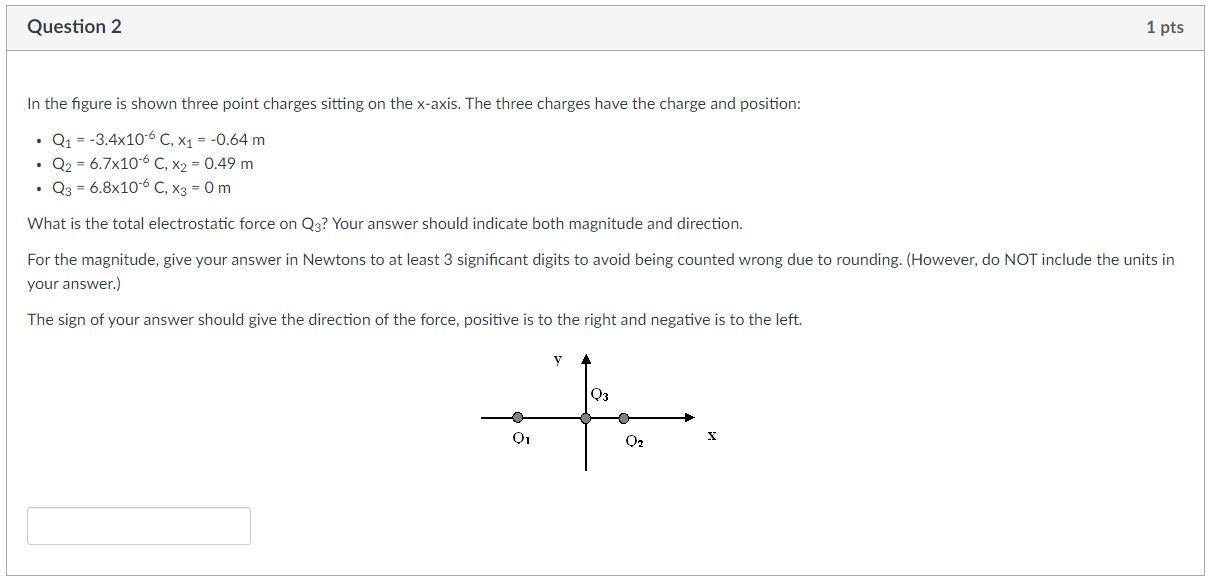Home / Expert Answers / Physics / in-the-figure-is-shown-three-point-charges-sitting-on-the-x-axis-the-three-charges-have-the-pa629

# (Solved): In the figure is shown three point charges sitting on the $$x$$-axis. The three charges have the ...In the figure is shown three point charges sitting on the $$x$$-axis. The three charges have the charge and position: - $$Q_{1}=-3.4 \times 10^{-6} \mathrm{C}, \mathrm{x}_{1}=-0.64 \mathrm{~m}$$ - $$Q_{2}=6.7 \times 10^{-6} \mathrm{C}, \mathrm{x}_{2}=0.49 \mathrm{~m}$$ - $$Q_{3}=6.8 \times 10^{-6} \mathrm{C}, \mathrm{x}_{3}=0 \mathrm{~m}$$ What is the total electrostatic force on $$\mathrm{Q}_{3}$$ ? Your answer should indicate both magnitude and direction. For the magnitude, give your answer in Newtons to at least 3 significant digits to avoid being counted wrong due to rounding. (However, do NOT include the units in your answer.) The sign of your answer should give the direction of the force, positive is to the right and negative is to the left.

We have an Answer from Expert